Library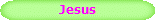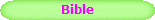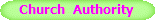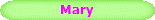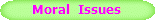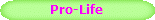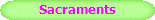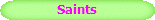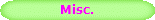DEFENDING  THE  BRIDE

Print Free Pamphlet - - Brief Summary

Why  153  Fish  in  John  21:11  ?

Sections :

Calculating the Measure of the Fish

John  the  Fisherman

Before John was called by Jesus to be His Disciple and Apostle, John was a professional fisherman.  At the end of his Gospel he makes 8 references to the measure of a fish.  This would bring to the minds of the Greeks the following mathematical calculations.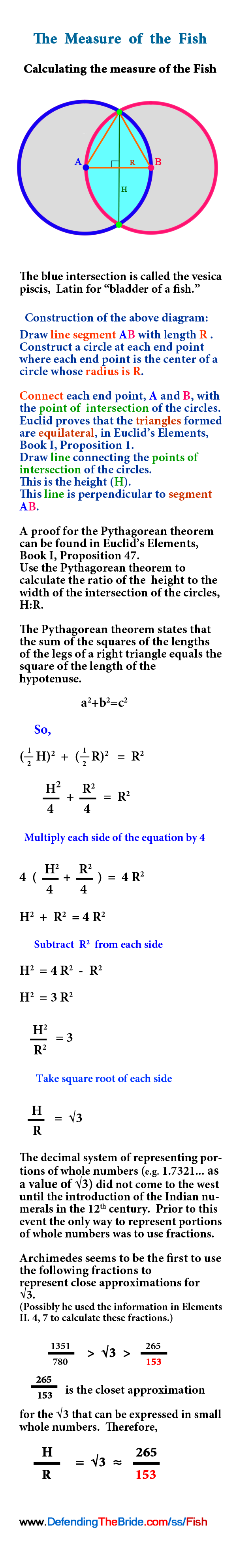Final Number

The square root of 3 is an irrational number.  If it was expressed in decimal form the numbers after the decimal point go on forever.  They do terminate and they do not repeat.  An approximation of  √3   would have to be used in a calculation if a rational answer was desired.  The decimal system did not come to the west until the 12th century so the approximation of   √3   would have to be expressed in a fractional, or ratio, format.  The horizontal fraction bar did not come to the west until the 12th century as well.  So, at the time John’s Gospel was written the rational approximation for the square root of   √3   would have be expressed in a linear, ratio, format as below.  The closest approximation of the square root of 3 expressed in small whole numbers and by its upper and lower limits is the following :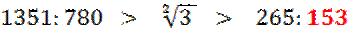Notice that the final number in this equation for the measure of the fish is the number  153.  John knew that his reference to the  fish,  and its measure together with his reference to the number 153 would not be lost upon the minds of the Greeks to whom he was writing.  John was referring to Greek wisdom specifically, and by extension to all wisdom.

So, the Greeks make the connections between the measure of the fish and the final number in the equation, 153.  It would also bring to their minds their great cultural heroes, Pythagoras, Euclid, and Archimedes.

Pythagoras and the Pythagoreans were best know for their Pythagorean Formula. And it was by this formula that the measure of the fish is determined.

Euclid was best known for his book on geometry, Elements.  And it is the first proposition of this book where he constructs the vesica piscis, which is the diagram of the fish.

Archimedes was known to have used the above approximation for square root of   √3  ending in the number 153.  (He does not explain from where he got this number.  So, possibly he was the first to use it.)

So, both the final number 153 in the answer to the question of
What is measure of the fish?,
as well as the method of how it is calculated would cause the Greeks of John's day to think of the quality of wisdom for which Pythagoras, Euclid, and Archimedes were highly esteemed.

Continue ...

Sections :

Calculating the Measure of the Fish

Print Free Pamphlet - - Brief Summary

NEW

See  Articles  at

JESUS     BIBLE     CHURCH   AUTHORITY

MARY     MORAL  ISSUES     PRO-LIFE

SACRAMENTS       SAINTS       MISC.

www.DefendingTheBride.com